## Parallelogram Martensite Microstructure

A martensitic twin involves fitting only two martensite variants together. Other microstructures are possible which use more than two variants, and one such is the parallelogram martensite microstructure. A parallelogram microstructure is formed when twins cross. Macroscopically, this microstructure appears as depicted in Figure 1 below.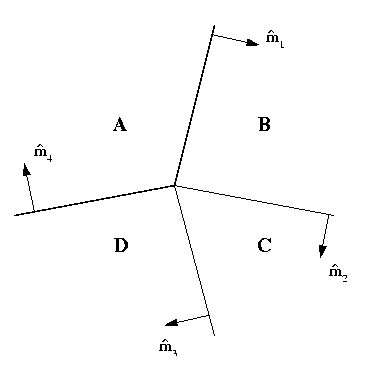Figure 1: Local parallelogram microstructure

Each of the deformation gradients, A, B, C, and D takes values from one of the martensite energy wells. They can be taken as A=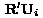, B=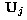, C=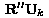, and D=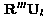, where the R's are rotations and the U's are variants of the martensite phase. Consequently, the compatibility equation for the parallelogram martensite microstructure can be written asEach of these compatibility equations is a twinning equation, and therefore the vectors b are parallel to the twin shears a, and the vectors m are parallel to the twin plane normals n.

Geometrically, the four planes with normals m's must intersect along a line; thus, they must be coplanar. Further, it can be shown that the twin shears b's must also be coplanar in order for all of the compatibility equations to be satisfied.

If the local compatibility equations are all satisfied, then the microstructure as depicted in Figure 1 can be repeated in a tiling pattern to fill all of the specimen as shown in Figure 2.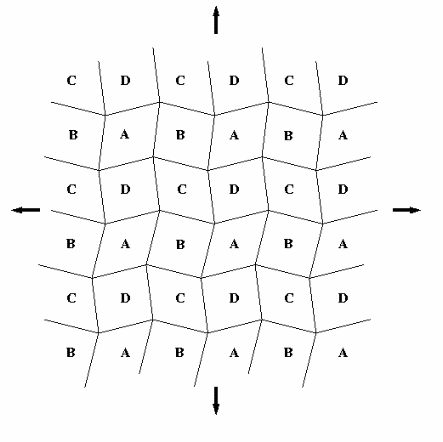Figure 2: Global parallelogram microstructure

Some of the parallelogram microstructures which are possible for the different transitions as listed in the table below.

#### Parallelogram Martensite Microstructures

Transition Twin Type Number Observed Comment
Cubic-to-Trigonal Compound 6 Au-Cd, Ti-Ni, Ti-Ni-Al, Ti-Ni-Fe possible for all trigonal angles
Cubic-to-Tetragonal Compound 0 No not possible for any lattice parameters
Cubic-to-Orthorhombic Compound and Type I 6 Cu-Al-Ni possible for all lattice parameters
Cubic-to-Monoclinic Compound 6 Ti-Ni possible for all lattice parameters

In the table above, the first column is the transition; the second gives the twins used in the construction; the third column lists the number of unique parallelogram martensite microstructures which can be formed using all of the variants of the martensite phase; and the fourth column gives alloys for which some of the twin crossings are observed. Moreover, for the cubic-to-trigonal, -orthorhombic and -monoclinic transitions, there may be other parallelogram microstructures.